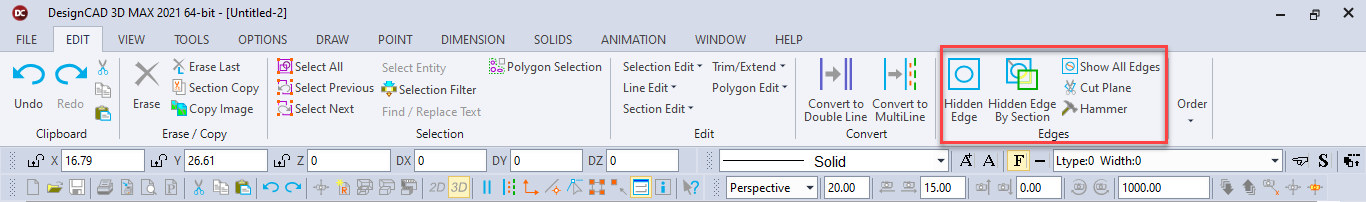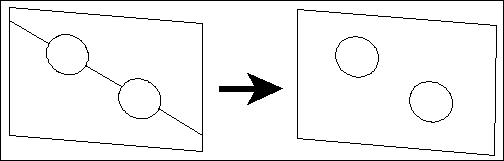# Hidden EdgeErases cut-plane lines which are sometimes a result of the Cut Plane, Polygon Subtract, and Solid Subtract commands.

After executing a Cut Plane, Polygon Subtract, or Solid Subtract command, select the Hidden Edge command. Set a point on one of the cut- plane lines so that it will be hidden from the view or from a printout of the drawing.

Example: Draw a large square plane and two small circular planes. Draw the circular planes so that they are contained by the square plane with respect to the XY plane. Use Polygon Subtract to subtract the circular planes from the square plane. Notice the cut-plane lines which are a necessity of this kind of operation. Choose the Hidden Edge command and set a point on one of the cut-plane lines. The cut-plane line will be removed from the view and will not be printed in subsequent printouts. Repeat the Hidden Edge command as many times as desired.